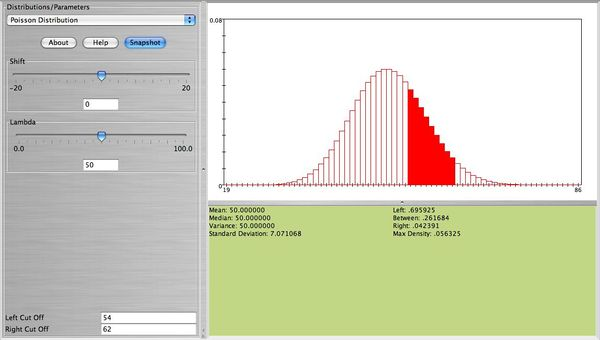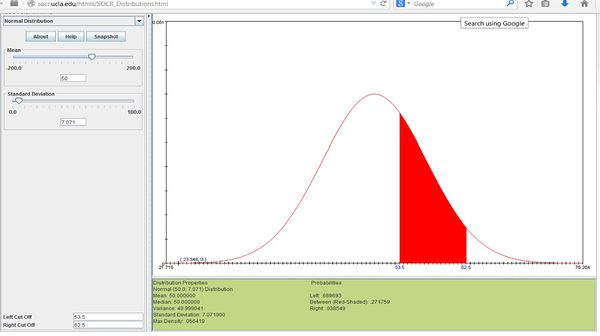# Difference between revisions of "AP Statistics Curriculum 2007 Limits Norm2Poisson"

## General Advance-Placement (AP) Statistics Curriculum - Normal Approximation to Poisson Distribution

### Normal Approximation to Poisson Distribution

The Poisson($$\lambda$$) Distribution can be approximated with Normal when $$\lambda$$ is large.

For sufficiently large values of λ, (say λ>1,000), the Normal($$\mu=\lambda, \sigma^2=\lambda$$) Distribution is an excellent approximation to the Poisson(λ) Distribution. If λ is greater than about 10, then the Normal Distribution is a good approximation if an appropriate continuity correction is performed.

If $$x_o$$ is a non-negative integer, $$X\sim Poisson(\lambda)$$ and $$U\sim Normal(\mu=\lambda, \sigma^2=\lambda$$), then $$P_X(X<x_o) = P_U(U<x_o+0.5)$$.

### Examples

Suppose cars arrive at a parking lot at a rate of 50 per hour. Let’s assume that the process is a Poisson random variable with $$\lambda=50$$. Compute the probability that in the next hour the number of cars that arrive at this parking lot will be between 54 and 62. We can compute this as follows$P(54 \le X \le 62) = \sum_{x=54}^{62} \frac{50^x e^{-50}}{x!}=0.2617.$ The figure below from SOCR shows this probability.• Note: We observe that this distribution is bell-shaped. We can use the normal distribution to approximate this probability. Using $$N(\mu=50, \sigma=\sqrt{50}=7.071)$$, together with the continuity correction for better approximation we obtain $$P(54 \le X \le 62)=0.2718$$, which is close to the exact that was found earlier. The figure below shows this probability.